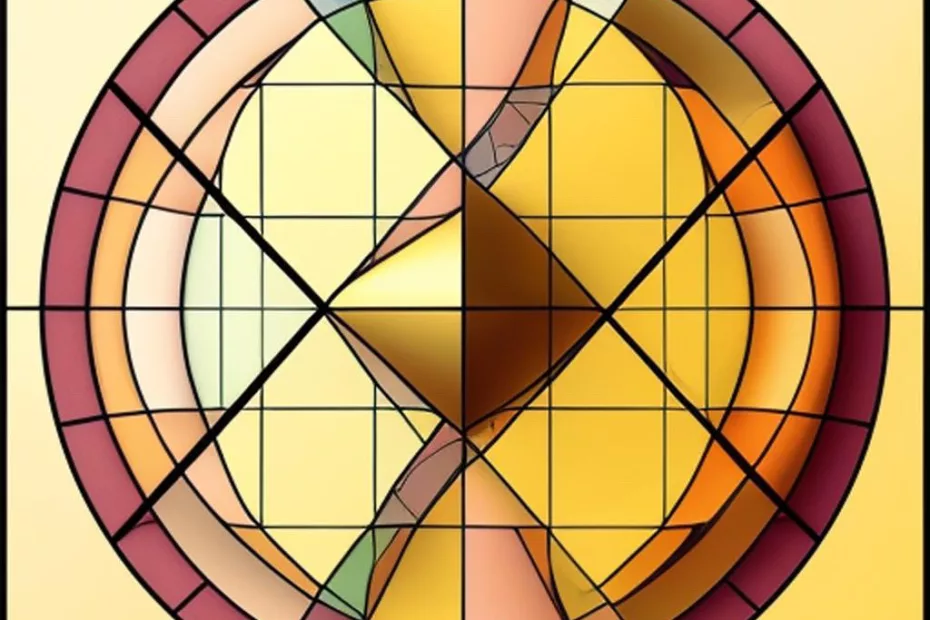# Understanding pi and the golden ratio through geometry rather than just abstract formalism

• AI TroT, Geometry, Mathematics## Understanding pi and the golden ratio through geometry rather than just abstract formalism

Let us highlight an important aspect of understanding mathematical concepts like pi and the golden ratio (golden number) through geometry rather than just abstract formalism. Geometry offers a tangible and visual representation of these mathematical constants, making them more accessible and intuitive to comprehend.

### Pi (π)

Pi is a mathematical constant that represents the ratio of a circle’s circumference to its diameter. While its decimal representation is non-terminating and non-repeating (approximately 3.14159…), its geometric significance is easily understood. In geometry, we see that the circumference of any circle is a little more than three times its diameter. This relationship is visual and intuitive, making the concept of pi more accessible even to those who might not be well-versed in advanced mathematics.

### Golden Ratio (Golden Number)

The golden ratio, often denoted by the Greek letter φ (phi), is an irrational number approximately equal to 1.6180339887… It has a unique mathematical property: when a line segment is divided into two parts in such a way that the ratio of the whole to the longer part is the same as the ratio of the longer part to the shorter part, the resulting ratio is the golden ratio. This ratio has aesthetic and harmonious qualities, and it appears in various aspects of art, architecture, and nature.

`Understanding the golden ratio often involves visually observing its presence in various geometric shapes and patterns, such as the golden rectangle and the Fibonacci spiral. The golden ratio's prevalence in art and nature can be seen in the dimensions of famous artworks, the arrangement of flower petals, and even the spiral patterns of seashells.`

Geometry provides an intuitive and experiential way to appreciate the beauty and significance of pi and the golden ratio. It allows individuals to directly visualize the relationships these constants represent, fostering a deeper understanding and appreciation for their mathematical properties.

While abstract mathematical formalism is a powerful tool for rigorous reasoning and proof, geometry serves as a bridge between mathematics and the physical world, offering a visual language that connects us to the underlying patterns and structures found in nature and the universe.

By exploring the geometric aspects of pi and the golden ratio, we not only gain a deeper understanding of these constants but also experience the profound interplay between mathematics and the world around us. This connection with the physical and visual realm enriches our understanding of mathematical concepts and their applications in diverse fields, from architecture and design to art and science.Understanding pi and the golden ratio through geometry rather than just abstract formalism

## Thank you for questions, shares and comments! 👍